• 主要介绍了关于tf.matmul() 和tf.multiply() 的区别说明，具有很好的参考价值，希望对大家有所帮助。一起跟随小编过来看看吧
• tf.multiplytf.matmul的区别 tf.multiply（）两个矩阵中对应元素各自相乘 tf.matmul（）将矩阵a乘以矩阵b，生成a * b import tensorflow as tf #两个矩阵的对应元素各自相乘！！ x=tf.constant([[1.0,2.0,3.0],...
np.random.rand(d0,d1,d2……dn)
通过本函数可以返回一个或一组服从“0~1”均匀分布的随机样本值。随机样本取值范围是[0,1)，不包括1。 1)当函数括号内没有参数时，则返回一个浮点数； 2）当函数括号内有一个参数时，则返回秩为1的数组，不能表示向量和矩阵； 3）当函数括号内有两个及以上参数时，则返回对应维度的数组，能表示向量或矩阵；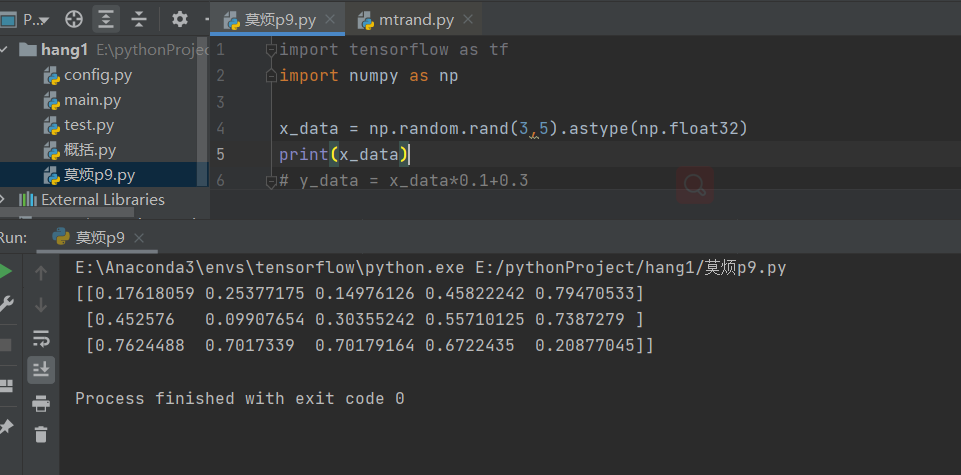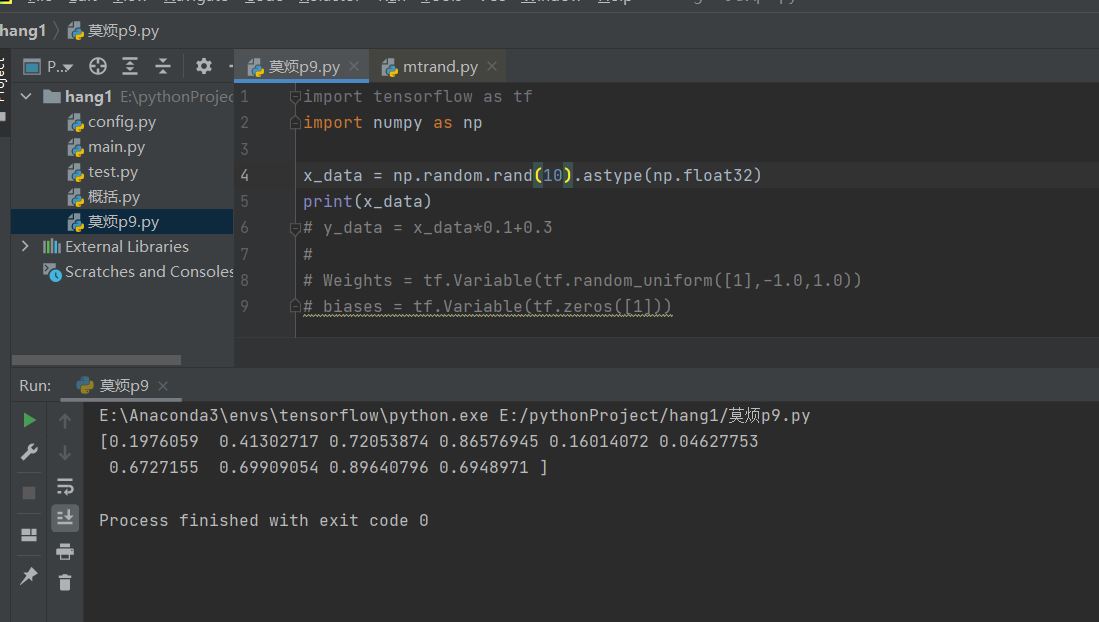newaxis
运行如下代码：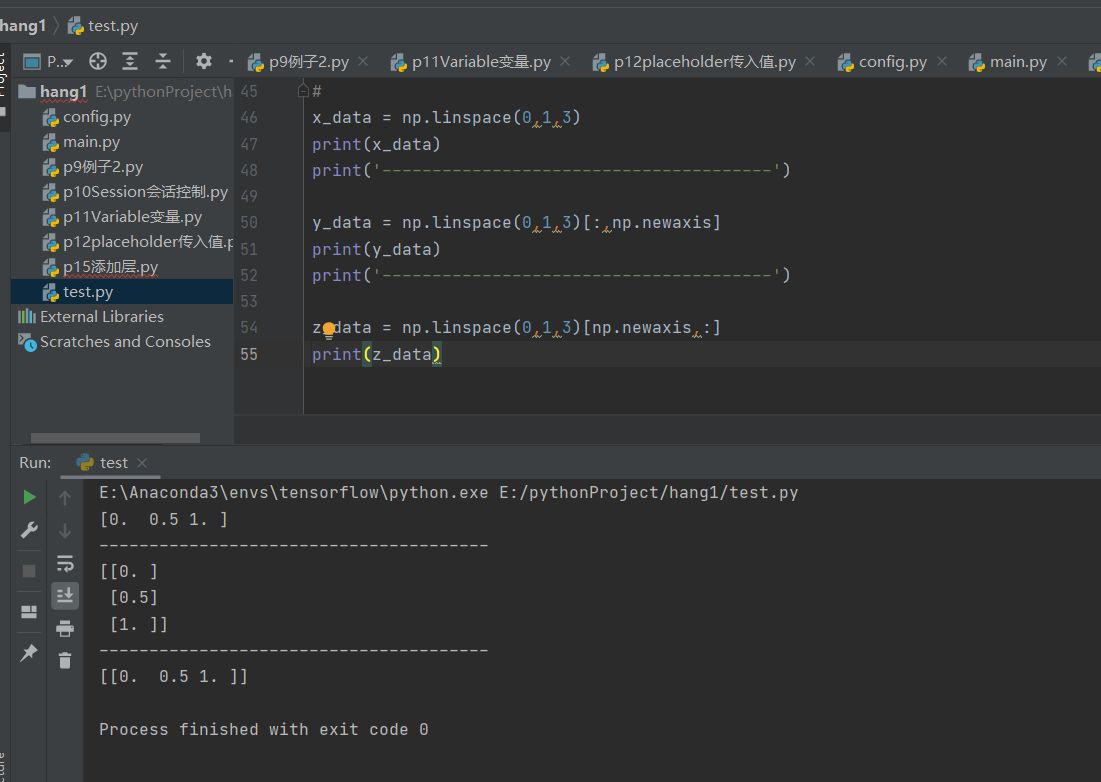由以上代码可以看出，以前的shape是3把newaxis放后面的时候，输出的新数组的shape就是3××1，也就是后面增加了一个维数newaxis把newaxis放在前面的时候变成了1××3，也就是前面的维数增加了一个newaxis放在第几个位置，就会在shape里面看到相应的位置增加了一个维数
tf.multiply与tf.matmul的区别
tf.multiply（）可用作两个矩阵中对应元素各自相乘等tf.matmul（）将矩阵a乘以矩阵b，生成a * btf.multiply不一定是逐个元素对应相乘，也可能是利用广播特性。
tf.multiply（）
import tensorflow as tf

#两个矩阵的对应元素各自相乘！！
x=tf.constant([[1.0,2.0,3.0],[1.0,2.0,3.0],[1.0,2.0,3.0]])
y=tf.constant([[0,0,1.0],[0,0,1.0],[0,0,1.0]])
#注意这里这里x,y要有相同的数据类型，不然就会因为数据类型不匹配而出错
z=tf.multiply(x,y)

#两个数相乘
x1=tf.constant(1)
y1=tf.constant(2)
#注意这里这里x1,y1要有相同的数据类型，不然就会因为数据类型不匹配而出错
z1=tf.multiply(x1,y1)

#数和矩阵相乘
x2=tf.constant([[1.0,2.0,3.0],[1.0,2.0,3.0],[1.0,2.0,3.0]])
y2=tf.constant(2.0)
#注意这里这里x1,y1要有相同的数据类型，不然就会因为数据类型不匹配而出错
z2=tf.multiply(x2,y2)
with tf.Session() as sess:
print(sess.run(z))
print(sess.run(z1))
print(sess.run(z2))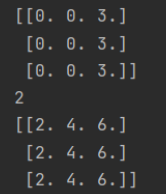tf.matmul（）
#两个矩阵相乘
x3=tf.constant([[1.0,2.0,3.0],[1.0,2.0,3.0],[1.0,2.0,3.0]])
y3=tf.constant([[0,0,1.0],[0,0,1.0],[0,0,1.0]])
#注意这里这里x,y要满足矩阵相乘的格式要求。
z3=tf.matmul(x,y)

with tf.Session() as sess:
print(sess.run(z3))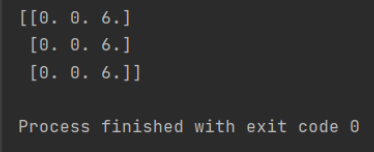tf.reduce_sum()函数和tf.reduce_mean()函数
tf.reduce_sum()
tf.reduce_sum
matrix1 = [[1.,2.,3.],            #二维，元素为列表
[4.,5.,6.]]
matrix2 = [[[1.,2.],[3.,4.]],      #三维，元素为矩阵
[[5.,6.],[7.,8.]]]

res_2 = tf.reduce_sum(matrix1)
res_3 = tf.reduce_sum(matrix2)
res1_2 = tf.reduce_sum(matrix1,reduction_indices=)
res1_3 = tf.reduce_sum(matrix2,reduction_indices=)
res2_2 = tf.reduce_sum(matrix1,reduction_indices=)
res2_3 = tf.reduce_sum(matrix2,reduction_indices=)

sess = tf.Session()
print("reduction_indices=None：res_2={}，res_3={}".format(sess.run(res_2),sess.run(res_3)))
print("reduction_indices=：res1_2={}，res1_3={}".format(sess.run(res1_2),sess.run(res1_3)))
print("reduction_indices=：res2_2={}，res2_3={}".format(sess.run(res2_2),sess.run(res2_3)))


result:
axis=None：res_2=21.0，res_3=36.0
axis=：res1_2=[5. 7. 9.]，res1_3=[[ 6.  8.]
[10. 12.]]
axis=：res2_2=[ 6. 15.]，res2_3=[[ 4.  6.]
[12. 14.]]


tf.reduce_mean 只需要把上面代码的reduce_sum部分换成renduce_mean即可
res_2 = tf.reduce_mean(matrix1)
res_3 = tf.reduce_mean(matrix2)
res1_2 = tf.reduce_mean(matrix1,axis=)
res1_3 = tf.reduce_mean(matrix2,axis=)
res2_2 = tf.reduce_mean(matrix1,axis=)
res2_3 = tf.reduce_mean(matrix2,axis=)


result:
axis=None：res_2=3.5，res_3=4.5
axis=：res1_2=[2.5 3.5 4.5]，res1_3=[[3. 4.]
[5. 6.]]
axis=：res2_2=[2. 5.]，res2_3=[[2. 3.]
[6. 7.]]


可以看到，reduction_indices和axis其实都是代表维度，当为None时，reduce_sum和reduce_mean对所有元素进行操作，当为时，其实就是按行操作，当为时，就是按列操作，对于三维情况，把最里面的括号当成是一个数，这样就可以用二维的情况代替，最后得到的结果都是在原来的基础上降一维，
Reference
1.终于弄懂tf.reduce_sum()函数和tf.reduce_mean()函数
2.tf.multiply与tf.matmul的区别
3.Numpy学习—np.random.randn()、np.random.rand()和np.random.randint()
展开全文• 1. tf.multiply 2. tf.matmul 3. tf.tensordot 1. tf.multiply tf.multiply 等同与 * ，用于计算矩阵之间的 element-wise 乘法，要求矩阵的形状必须一致(或者是其中一个维度为1)，否则会报错。 import ...
1. tf.multiply
2. tf.matmul
3. tf.tensordot

1. tf.multiply
tf.multiply 等同与 * ，用于计算矩阵之间的 element-wise 乘法，要求矩阵的形状必须一致(或者是其中一个维度为1)，否则会报错。
import tensorflow as tf

a = tf.constant([1, 2, 3, 4, 5, 6, 7, 8, 9, 10,11,12], shape=[2, 3, 2])
b = tf.constant([1, 2, 3, 4, 5, 6], shape=[2, 3, 1])

c = a * b
d = tf.multiply(a, a)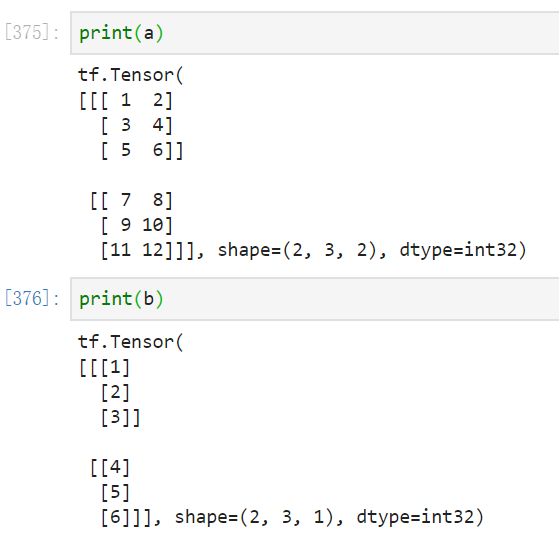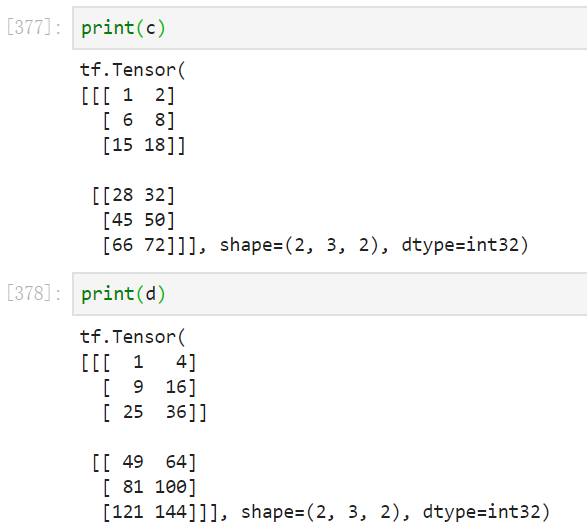2. tf.matmul
tf.matmul 是 tensor 的矩阵乘法，参与运算的两个tensor维度、数据类型必须一致。
a = tf.constant(list(range(1, 13)), shape=[3, 4])
b = tf.constant(list(range(1, 13)), shape=[4, 3])

c = tf.matmul(a, b)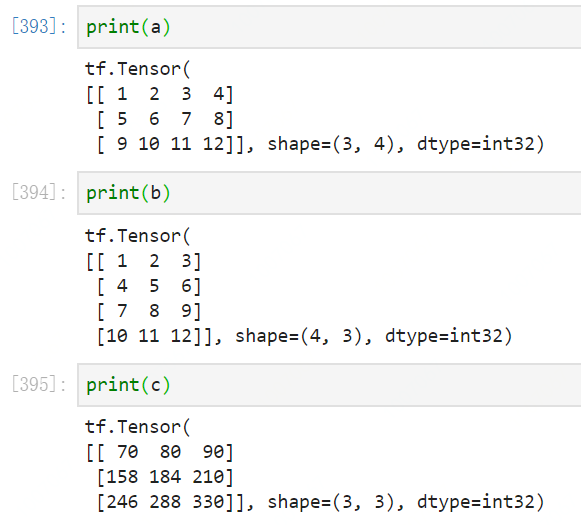3. tf.tensordot
tensordot：矩阵乘法运算，参与运算的两个tensor的维度可以不一样。
a 和 b 沿特定轴的张量收缩。 Tensordot (也称为张量收缩) 对从 a 和 b 所指定的索引 a_axes 和 b_axes 的元素的乘积进行求和。 列表 a_axes 和 b_axes 指定沿其收缩张量的那些轴对。 对于所有 range(0, len(a_axes)) 中的 i，a 的轴 a_axes[i] 必须与 b 的轴 b_axes[i] 具有相同的维度。 列表 a_axes 和 b_axes 必须具有相同的长度，并由唯一的整数组成，用于为每个张量指定有效的坐标轴。
该操作对应于 numpy.tensordot(a, b, axes) 示例1：当 a 和 b 是矩阵(2阶)时，axes = 1 相当于矩阵乘法； 示例2：当 a 和 b 是矩阵(2阶)时，axes = [, ] 相当于矩阵乘法；
函数参数： • a：float32 或 float64 类型的 Tensor； • b：Tensor，与 a 具有相同的类型； • axes：可以是标量 N，也可以是具有形状 [2, k] 的 int32 Tensor 的列表。如果轴是标量，则按顺序对 a 的最后 N 个轴和 b 的前 N 个轴进行求和。         如果轴是一个列表或 Tensor，则分别对于轴 a 和 b，在第一和第二行包含该组唯一整数指定沿该收缩被计算。a 和 b 的坐标轴数必须相等； • name：操作的名称(可选)；
函数返回值： 函数返回与 a 具有相同类型的 Tensor；
可能引发的异常： • ValueError：如果 a, b 和 axes 的形状是不相容的； • IndexError：如果轴上的值超过相应张量的等级；

a = tf.constant([1, 2, 3, 4, 5, 6, 7, 8, 9, 10,
11, 12, 13, 14, 15, 16, 17, 18, 19, 20,
21, 22, 23, 24], shape=[2, 3, 4])

b = tf.constant([1, 2, 3, 4, 5, 6, 7, 8, 9, 10, 11, 12], shape=[4, 3])

c = tf.tensordot(a, b, axes=1)
# 同 tf.matmul，普通矩阵相乘
# shape: [2, 3, 4] x [4, 3] = [2, 3, 3]

d = tf.tensordot(a, b, axes=2)
# 对a的后2个轴的数据进行Flatten，即a的shape变成 [2, 3X4] = [2, 12];
# 对b的前2个轴的数据进行Flatten，即b的shape变成
# shape: [2, 12] x  = [2,]

e = tf.tensordot(a, b, axes=([1,2], [0,1]))
# 分别指定两个轴，此结果同 tf.tensordot(a, b, axes=2)
# 对a的后2个轴（索引1，2）的数据进行Flatten，即a的shape变成 [2, 3X4] = [2, 12];
# 对b的前2个轴（索引0，1）的数据进行Flatten，即b的shape变成，展开结果[1,2,3,4,5,6,7,8,9,10,11,12]
# shape: [2, 12] x  = [2,]

f = tf.tensordot(a, b, axes=([1,2], [1,0]))
# 分别指定两个轴
# 对a的后2个轴（索引1，2）的数据进行Flatten，即a的shape变成 [2, 3X4] = [2, 12];
# 对b的前2个轴（索引1，0）的数据进行Flatten，即b的shape变成，轴的顺序不同，展开方式不同，展开结果[1,4,7,10,2,5,8,11,3,6,9,12]
# shape: [2, 12] x  = [2,]

g = tf.tensordot(a, b, axes=(, ))
# 指定任何轴，指定的轴形状一致
# shape: [2, 3, 4] x [4, 3] = [2, 4, 4] （消去（3，3））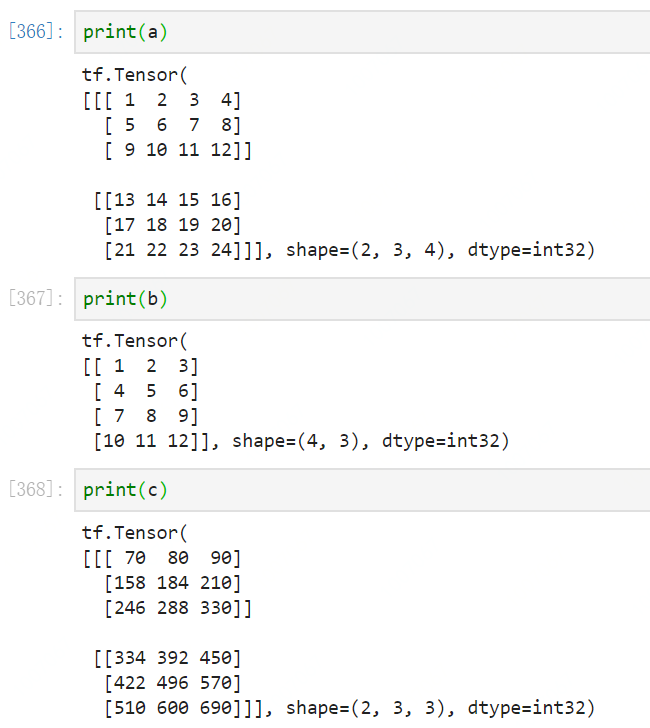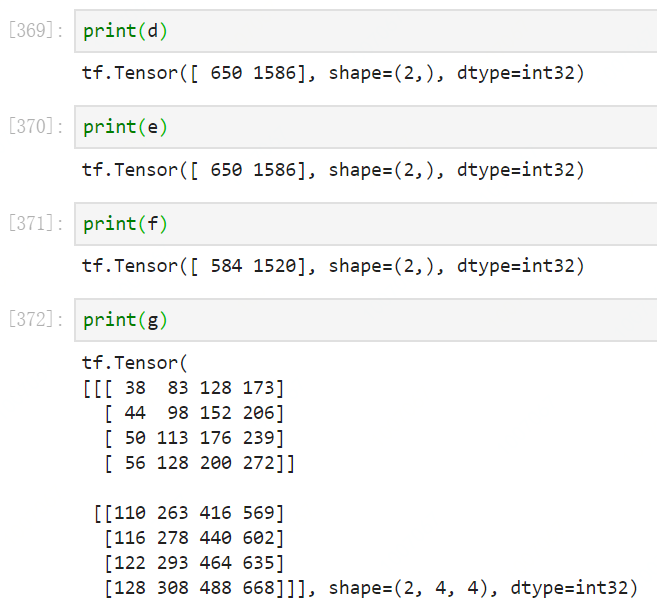展开全文• tf.multiply : 按元素相乘 # [64*6*30, 2] * [64*6*30,1] this_platf_target_outputs = tf.multiply(neighbor_part_inputs, mask[target_node, platf, ...]) Returns x * y element-wise tf.matmul: 矩阵相乘 ...
tf.multiply : 按元素相乘 等于torch.mul()
 # [64*6*30, 2] * [64*6*30,1]
this_platf_target_outputs = tf.multiply(neighbor_part_inputs, mask[target_node, platf, ...])


Returns x * y element-wise

tf.matmul: 矩阵相乘
this_platf_target_outputs = tf.matmul(this_platf_target_outputs, self.wc[platf]) + self.bc[platf]  # shape=(seq_len*num_nodes, units)


展开全文• tf.add()、tf.subtract()、tf.multiply()、tf.div()函数介绍和示例 1. tf.add() 释义：加法操作 示例： x = tf.constant(2, dtype=tf.float32, name=None) y = tf.constant(3, dtype=tf.float32, name=None) z = tf....
tf.add()、tf.subtract()、tf.multiply()、tf.div()函数介绍和示例
释义：加法操作
示例：
x = tf.constant(2, dtype=tf.float32, name=None)
y = tf.constant(3, dtype=tf.float32, name=None)
z = tf.add(x, y)         # 加法操作

X = tf.constant([[1, 2, 3], [4, 5, 6]], dtype=tf.float32, name=None)
Y = tf.constant([[1, 1, 1], [2, 2 ,2]], dtype=tf.float32, name=None)
Z = tf.add(X, Y)         # 矩阵加法操作，对应位置元素相加

with tf.Session() as sess:
print(sess.run(z))
print('='*30)

print(sess.run(Z))

5.0
==============================
[[2. 3. 4.]
[6. 7. 8.]]

2. tf.subtract()
释义：减法操作
示例：
x = tf.constant(10, dtype=tf.float32, name=None)
y = tf.constant(4, dtype=tf.float32, name=None)
z = tf.subtract(x, y)         # 减法操作

X = tf.constant([[1, 2, 3], [4, 5, 6]], dtype=tf.float32, name=None)
Y = tf.constant([[1, 1, 1], [2, 2 ,2]], dtype=tf.float32, name=None)
Z = tf.subtract(X, Y)         # 矩阵减法操作，对应位置元素相减

with tf.Session() as sess:
print(sess.run(z))
print('='*30)

print(sess.run(Z))

6.0
==============================
[[0. 1. 2.]
[2. 3. 4.]]

3. tf.multiply()
释义：将两个矩阵中对应元素各自相乘
示例：
import tensorflow as tf

X = tf.constant([[1, 2, 3], [4, 5 ,6]], dtype=tf.float32, name=None)
Y = tf.constant([[1, 1, 1], [2, 2 ,2]], dtype=tf.float32, name=None)
Z = tf.multiply(X, Y)       # 乘法操作，对应位置元素相乘

with tf.Session() as sess:
print(sess.run(Z))

[[ 1.  2.  3.]
[ 8. 10. 12.]]

tf.matmul()和tf.scalar_mul()函数介绍和示例见csdn 博客
4. tf.div()
释义：除法操作
示例：
x = tf.constant(6, dtype=tf.float32, name=None)
y = tf.constant(3, dtype=tf.float32, name=None)
z = tf.div(x, y)           # 标量/标量

X1 = tf.constant(6, dtype=tf.float32, name=None)
Y1 = tf.constant([[1, 2], [2, 3]], dtype=tf.float32, name=None)
Z1 = tf.div(X1, Y1)        # 标量/矩阵

X2 = tf.constant([[6, 12], [6, 12]], dtype=tf.float32, name=None)
Y2 = tf.constant([[1, 2], [2, 3]], dtype=tf.float32, name=None)
Z2 = tf.div(X2, Y2)        # 矩阵/矩阵，对应元素相除

with tf.Session() as sess:
print(sess.run(z))
print('='*30)

print(sess.run(Z1))
print('='*30)

print(sess.run(Z2))

2.0
==============================
[[6. 3.]
[3. 2.]]
==============================
[[6. 6.]
[3. 4.]]


展开全文tensorflow
• 三、tf.multiply()理解 一、tf.reduce_mean 函数 用于计算张量tensor沿着指定的数轴（tensor的某一维度）上的的平均值，主要用作降维或者计算tensor（图像）的平均值，默认对所有的元素求平均。 第一个参数input_...
• tf.multiply()、tf.matmul()、tf.scalar_mul()函数介绍和示例 1. tf.multiply() 释义：将两个矩阵中对应元素各自相乘 示例： import tensorflow as tf X = tf.constant([[1, 2, 3], [4, 5 ,6]], dtype=tf.float32, ...tensorflow
• import tensorflow as tf #两个矩阵的对应元素各自相乘！！ x=tf.constant([[1.0,2.0,3.0],[1.0,2.0,3.0],[1.0,2.0,3.0]]) y=tf.constant([[0,0,1.0],[0,0,1.0],[0,0,1.0]]) #注意这里这里x,y要有相同的数据类型...
• multiply 等同与* ，用于计算矩阵之间的element-wise 乘法，要求矩阵的形状必须一致(或者是其中一个维度为1)，否则会报错： import tensorflow as tf a = tf.constant([1, 2, 3, 4, 5, 6, 7, 8, 9, 10,11,12], ...
• tf.multiply(x, y, name=None) np.multiplytf.multiply用法一致，可以放在一起理解。功能是实现x和y的element-wise方式的相乘，也就是所有点对应相乘。注意不是矩阵乘操作，矩阵乘操作使用的是tf.matmul函数。 ...
• tf.matmul矩阵乘法，叉乘。 tf.multiply和*一样都是点乘，对应位置的元素相乘。
• 1.tf.multiply（）两个矩阵中对应元素各自相乘 格式:tf.multiply(x, y, name=None) 参数: x: 一个类型为:half, float32, float64, uint8, int8, uint16, int16, int32, int64, complex64, complex128的张量。 y: 一...
• tf.math.multiply( x, y, name=None ) 输入： x:一个tensor，类型得是bfloat16, half, float32, float64, uint8, int8, uint16, int16, int32, int64, complex64, complex128. y:跟x同类型。 输出： 逐个元素...tensorflow
• 目标： 两个相同大小的矩阵，相同位置上的元素对应各自相乘 Pytorch语法： torch.mul(tensorA, tensorB) Tensorflow语法： tf.multiply(tensorA, tensorB) 示例：tensorflow pytorch
• import tensorflow as tf import numpy as np x1 = ([[1, 2, 3], [1, 2, 3], [1, 2, 3]]) x2 = ([[2, 1, 1], [2, 1, 1], [2, 1, 1]]) y1 = np.dot(x1, x2) y2 = np.multiply(x1, x2) print('1、np.dot\n', y1) ...tensorflow numpy matmul
• **tf.multiply（）**两个矩阵中对应元素各自相乘 格式: tf.multiply(x, y, name=None) 参数: x: 一个类型为:half, float32, float64, uint8, int8, uint16, int16, int32, int64, complex64, complex128的...tensorflow
• # a ...# [4, 5, 6]] a = tf.constant([1, 2, 3, 4, 5, 6], shape=[2, 3]) # b1 # [[ 7, 8], # [ 9, 10], # [11, 12]] b1 = tf.constant([7, 8, 9, 10, 11, 12], shape=[3, 2]) #b2 #[[ 7 8 9] ...
• tf.matmul（）是常见的矩阵相乘运算，而tf.multiply（）则是两个矩阵中对应元素的相乘运算。 具体用法： multiply 等同与* ，用于计算矩阵之间的element-wise 乘法，要求矩阵的形状必须一致(或者是其中一个维度为1...
• tf.multiply： 函数原型： tf.math.multiply( x, y, name=None ) 说明： 作用：对应元素相乘，并且具有广播作用。 x: 类型为:half, float32, float64, uint8, int8, uint16, int16, int32, int64, complex64, ...
• tf.multiply()这个是相乘的方法，如果是单纯的数字的话，很简单就是直接乘 如果是矩阵呢？ 必须是同一维度的两个矩阵相乘 相乘就是对应位置的数相乘 1*1=1 2*3=6 1*!=1 1*2=2 5*4=20 1*2=2 ...
• tf.multiply(x,y,name=None) 乘法，相同位置的元素相乘 x,y要有相同的数据类型 half, float32, float64, uint8, int8, uint16, int16, int32, int64, complex64, complex128. 例如 x =...
• 这个结果是怎么算出来的？ 教科书告诉你，计算规则是，第一个矩阵第一行的每个数字（2和1），各自乘以第二个矩阵第一列对应位置的数字（1和1），然后将乘积相加（ 2 x 1 + 1 x 1），得到结果矩阵左上角的那个值3。...
• tf.matmul() 和tf.multiply() 的区别 tf.multiply（）两个矩阵中对应元素各自相乘 tf.matmul（）将矩阵a乘以矩阵b，生成a * b。 https://www.cnblogs.com/AlvinSui/p/8987707.html python广播 ...可视化模块GraphViz’s......